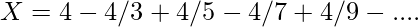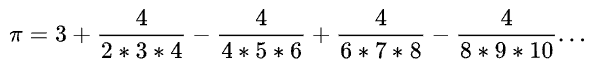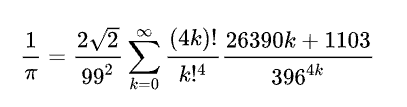×

Search anything:

# Different ways to calculate Pi (3.14159...)

#### Algorithms List of Mathematical AlgorithmsIn this article, we have covered different algorithms and approaches to calculate the mathematical constant pi (3.14159...). These include Nilakantha Series, Leibniz’s Formula, Ramanujan's Pi Formula and other Programming Language specific techniques.

1. Method 1: Leibniz’s Formula
2. Method 2: Nilakantha Series
3. Method 3: Ramanujan's Pi Formula
4. Method 4: Function acos()
5. Method 5: Math module
6. Method 6: gmpy module

We will get started with Different ways to calculate Pi (3.14159...).

# Method 1: Leibniz’s Formula

This equation can be implementd in any programming language.
Accuracy of value of pie depends on number of terms present in the equation which means high number of iterations produce better result.### Implementation of Leibniz’s Formula:

1. We will create 2 variables sum, d (denominator)
2. Initialise `sum = 0`
3. Initialise `d = 1`
4. Create a for loop
5. Loop i from 0 to 1000000 ( bigger number = more precsion )
6. Check if i is even then `sum=sum+4/d`, else `sum=sum-4/d`
7. Increment d by 2 every at every iteration
8. Print sum

### Time Complexity:

O(N * logN * loglogN), where

• N = number of iterations
• Division of two numbers of order O(N) takes O(logN loglogN) time.

### Code:

``````    #Initialize denominator
d = 1
#Initialize sum
sum = 0

for i in range(0,1000000):

if i % 2 == 0:
sum + = 4/d
else:
#odd index subtract from sum
sum -= 4/d

#increment denominator by 2
d += 2

print("Value of Pi is : ",sum)
``````

### Sample Output:

``````   Value of Pi is : 3.1415916535897743
``````

# Method 2: Nilakantha Series

It is somewhat similar to the previous method and also one of the conventional methods. As number of iterations increases the value of pi also gets precise.### Implementation:

1. Create 3 variables n,sum,sign
2. Initialse `sum=3`, `n=2`, `sign=1`
3. Create a for loop
4. loop from 0 to 1000000
5. At every iteration multiply `sign=sign*(-1)`
6. Calculate `sum=sum+sign*(4/(n)*(n+1)*(n+2))`
7. Increment n by 2 at every iteration
8. Print sum

### Time Complexity:

O(N * logN * loglogN), where

• N = number of iterations
• Time Complexity of multiplication and division is O(logN loglogN) at the best and O(logN logN) in general.

### Code:

``````   #Initialise sum=3,n=2,and sign=1
sum=3
n=2
sign=1

for i in range(0,1000000):

sum=sum+(sign*(4/((n)*(n+1)*(n+2))))

#for addition and subtraction of alternate terms
sign=sign*(-1)

#Increment by 2 according to formula
n=n+2

print("Value of Pi is : ",sum)
``````

### Sample Output:

``````   Value of Pi is : 3.141592653589787
``````

# Method 3: Ramanujan's Pi Formula

Ramanujan's Pi formula is one of the best methods to find numerical approximation of pi in less number of iterations. It may look difficult to implement but that is not the case, it's pretty simple, just follow these steps.### Implementation:

1. Firstly import math module
2. Create function to calculate factorial
3. Create function to calculate Pi by Ramanujan's Formula
4. Initialise `sum=0`,`n=0`,`i=math.sqrt(8)/9801`
5. Start the infinite while loop
6. Apply Ramanujan's Formula
7. Add the value to `sum+=tmp`
8. At every iteration increase n by 1, `n=n+1`
9. If the value has reached femto level that is 15th digit break the loop
10. Return 1/sum according to the formula
11. Print the value of sum

### Time Complexity:

O(N2 logN loglogN), where

• N = number of iterations
• Time Complexity of multiplication and division is O(logN loglogN) at the best and O(logN logN) in general.

Though the Time Complexity is higher than previous approaches, in this approach, one will need significantly less number of iterations so this is considered to be an effective technique.

### Code

``````   import math

#finds factorial for given number
def factorial(x):
if x==0:
return 1
else:
return r = x*factorial(x-1)

#Ramanujan formula for pi calculation
def ramanujan_pi():

#initialise sum=0, n=0 , and a variable i  to store 2√2/(99)^2
sum = 0
n = 0
i = (math.sqrt(8))/9801

while True:
#Ramanujan's Formula:-
tmp = i*(factorial(4*n)/pow(factorial(n),4))   ((26390*n+1103)/pow(396,4*n))
sum +=tmp

#Stop loop when it reaches 15th digit precision (femto)
if(abs(tmp) < 1e-15):
break
n += 1

return(1/sum)

print("Vaule of Pi is : ",ramanujan_pi())
``````

### Sample Output

``````   Vaule of Pi is : 3.141592653589793
``````

# Method 4: Function acos()

acos() is an inbuilt function in C++ STL and is also present python language and it’s the same as the inverse of cosine in maths. The acos() function returns the values in the range of [-π,π] that is an angle in radian. It can only show till 15th digit precison.

### Implementation

1. Import acos from math library
2. Define the function to take input n the number of decimal places
3. Use round function to get the pi value to desired decimal place
4. Use formula `pi=round(2*acos(0.0),n)`
5. Print value of by calling the function pi

O(1)

### Code:

``````   #Import acos from math
from math import acos

#define function
def valueofpi(n):

#Calculates pi with precision n, where n<=15
pi=round(2*acos(0.0),n)

#prints pi with n precision
print(pi)

print("Value of Pi is : ",valueofpi(15))

``````

### Sample Output:

``````   Value of Pi is : 3.141592653589793
``````

# Method 5: Math module

This is one of the simplest method to get the value of Pi without much hassle, it saves a lot of time.
Directly get the value of pi by using math module in python.
One drawback is that you can not get as precise result as the previous methods would provide.

O(1)

### Code:

``````   #import math module
import math

#print value
print("Value of Pi is : ",math.pi )

``````

### Sample Output:

``````   Value of Pi is : 3.141592653589793
``````

# Method 6: gmpy module

This is the best option in most of the cases , you can directly get the value of pi upto your desired precison with this module.
It is the better version of math module and nmpy module for calculating pi.

O(1)

### Code:

``````   #import gmpy module
import gmpy

#print value upto 128 bit precision
#Note that it takes argument in bits not in decimal digits
print ("Value of Pi is : ",gmpy.pi(128))

``````

### Sample Output:

``````Value of Pi is : 3.1415926535897932384626433832795
``````

With this article at OpenGenus, you must have the complete idea of different approaches to find the value of Pi.#### Kartik Keyan Kant

B. Tech | CSE | 3rd year | C++ | Java | C | AI | Bangalore | inbuilt function __learning( )

Different ways to calculate Pi (3.14159...)